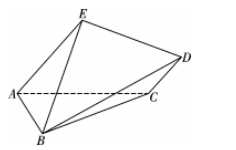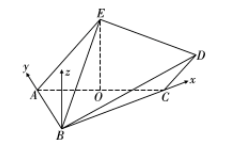(1) 若平面 $A B E \cap$ 平面 $B C D=l$, 求证: $l / /$ 平面 $A C D E$;
(2) 求平面 $A B E$ 与平面 $B C D$ 的锐二面角的余弦值.【答案】 (1) 证明: $A E / / C D, A E \subset$ 平面 $A B E, C D \not \subset$ 平面 $A B E \Rightarrow C D / /$ 平面 $A B E$,

$C D \subset$ 平面 $B C D$, 平面 $A B E \cap$ 平面 $B C D=l \Rightarrow C D / / l$,

$C D \subset$ 平面 $A C D E, l \not \subset$ 平面 $A C D E \Rightarrow l / /$ 平面 $A C D E$.

（2）解：由题意易知: $A B \perp B C, \angle C A E=60^{\circ}$, 如图, 设 $O$ 为 $A C$ 的中点,

$\Rightarrow O E \perp$ 平面 $A B C$.$$\overrightarrow{C D}=\frac{1}{2} \overrightarrow{A E}=(\sqrt{3},-1,2 \sqrt{3}) \Rightarrow D(5 \sqrt{3},-1,2 \sqrt{3}) \text {, }$$

$$\left\{\begin{array} { l } { 4 \sqrt { 3 } x = 0 } \\ { 5 \sqrt { 3 } x - y + 2 \sqrt { 3 } z = 0 } \end{array} \Rightarrow \left\{\begin{array}{l} x=0 \\ y=2 \sqrt{3} z \end{array} \Rightarrow \bar{m}=(0,2 \sqrt{3}, 1),\right.\right.$$

\begin{aligned} & \left\{\begin{array} { l } { 4 y = 0 } \\ { \sqrt { 3 } x + y + 2 \sqrt { 3 } z = 0 } \end{array} \Rightarrow \left\{\begin{array}{l} y=0 \\ x=-2 z \end{array} \Rightarrow \vec{n}=(-2,0,1),\right.\right. \\ & \Rightarrow \cos \left\langle\vec{m}, \vec{n} > =\frac{\vec{m} \cdot \vec{n}}{|\vec{m} \| \vec{n}|}=\frac{1}{\sqrt{13} \times \sqrt{5}}=\frac{\sqrt{65}}{65},\right. \end{aligned}